# 1 Introduction

Cardinal 3 provides statistical methods for both supervised and unsupervised analysis of mass spectrometry (MS) imaging experiments. Class comparison can also be performed, provided an appropriate experimental design and sample size.

Before statistical analysis, it is important to identify the statistical goal of the experiment:

• Unsupervised analysis. The data has no class labels or conditions, and we are interested in exploratory analysis to discover regions of interest in the data.

• Supervised analysis. The data has class labels and we want to train a statistical or machine learning model to predict the class labels of new data.

• Class comparison. The data has class labels or conditions, and we want to test whether the abundance of the mass features is different between conditions.

CardinalWorkflows provides real experimental data and more detailed discussion of the statistical methods than will be covered in this brief overview.

# 2 Exploratory analysis

Suppose we are exploring an unlabeled dataset, and wish to understand the structure of the data.

``````set.seed(2020)
mse <- simulateImage(preset=2, npeaks=10, dim=c(20,20), sdnoise=0.5,
peakheight=c(2,4), representation="centroid")

design <- makeFactor(circle=mse\$circle, square=mse\$square,
bg=!(mse\$circle | mse\$square))

image(mse, design ~ x * y, key=TRUE)``````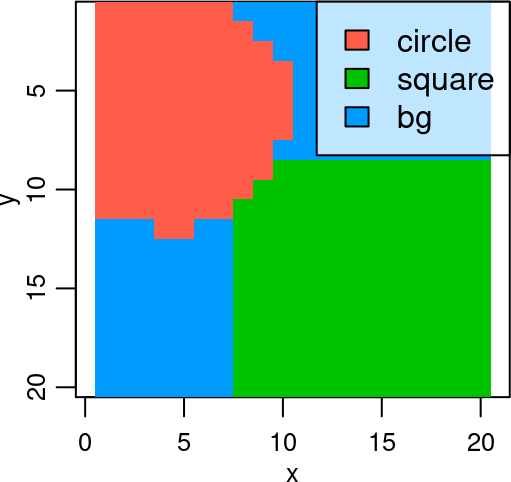``image(mse, feature=c(1,4,7), layout=c(1,3))``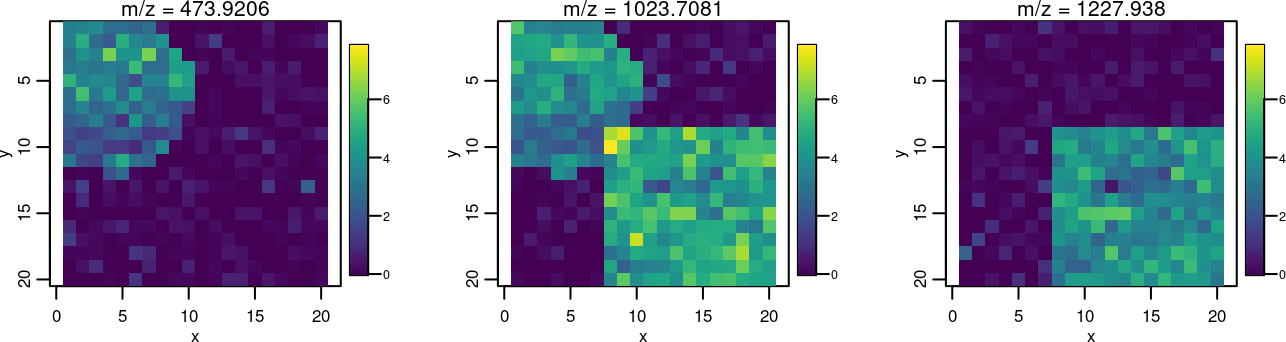## 2.1 Principal components analysis (PCA)

Principal components analysis is an unsupervised dimension reduction technique. It reduces the data to some number of “principal components” that are a linear combination of the original mass features, where each component is orthogonal to the last, and explains as much of the variance in the data as possible.

Use `PCA()` to perform PCA on a `MSImagingExperiment`.

``````pca <- PCA(mse, ncomp=3)

summary(pca)``````
``````## Principal components analysis:
##
##   Component Standard deviation
## 1         1          4.8523803
## 2         2          2.5724914
## 3         3          0.6767562``````

We can see that the first two principal components explain most of the variation in the data.

``image(pca, values="scores", superpose=FALSE, layout=c(1,3))``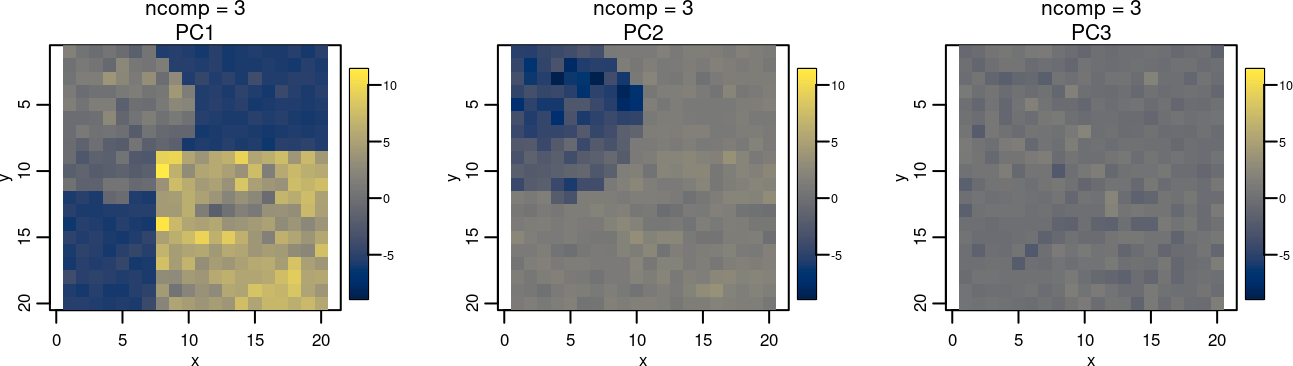The loadings of the components show how each mass feature contributes to each component.

``plot(pca, values="loadings", superpose=FALSE, layout=c(1,3), lwd=2)``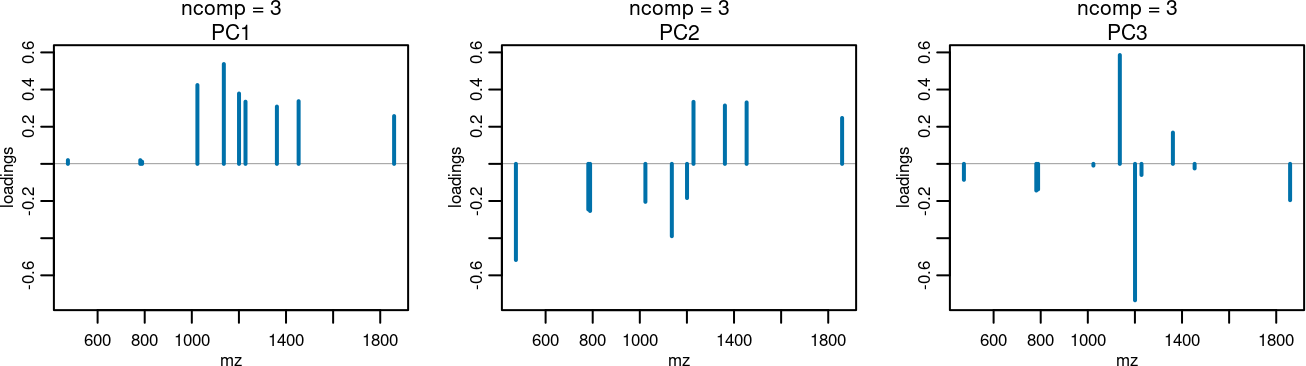Plotting the principal component scores against each other is a useful way of visualization the separation between data classes.

``````pca_scores <- DataFrame(resultData(pca, 1, "scores"))

plot(pca_scores, PC1 ~ PC2, groups=design, pch=20)``````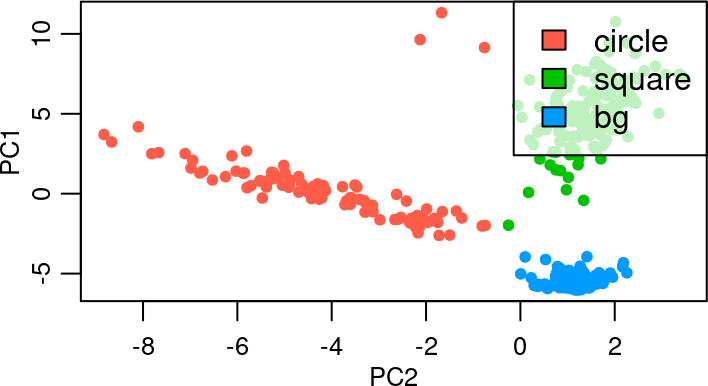## 2.2 Feature colocalization

Finding other mass features colocalized with a particular image is a common task in analysis of MS imaging experiments.

Use `colocalize()` to find mass features that are colocalized with another image.

``````coloc <- colocalized(mse, mz=1023)
coloc``````
``````## Colocalized features:
##           mz   circle   square correlation    M1    M2
## 1  1023.7081 2.011661 4.063644   1.0000000 1.000 1.000
## 2  1135.9335 2.434873 3.985370   0.9433140 0.910 0.910
## 3  1200.4653 2.219637 4.166854   0.9201669 0.900 0.900
## 4  1453.5096 0.000000 4.187344   0.7080514 0.770 0.770
## 5  1227.9380 0.000000 4.039750   0.7056904 0.750 0.750
## 6  1858.8985 0.000000 3.970513   0.6916683 0.765 0.765
## 7  1361.2682 0.000000 4.259568   0.6906648 0.760 0.760
## 8   473.9206 2.340799 0.000000   0.2984935 0.530 0.530
## 9   781.2367 1.392247 0.000000   0.2979227 0.575 0.575
## 10  788.8633 1.542205 0.000000   0.2713569 0.555 0.555``````

By default, Pearson correlation is used to rank the colocalized features. Manders’ colocalization coefficients (M1 and M2) are also provided.

``image(mse, mz=coloc\$mz[1:3], layout=c(1,3))``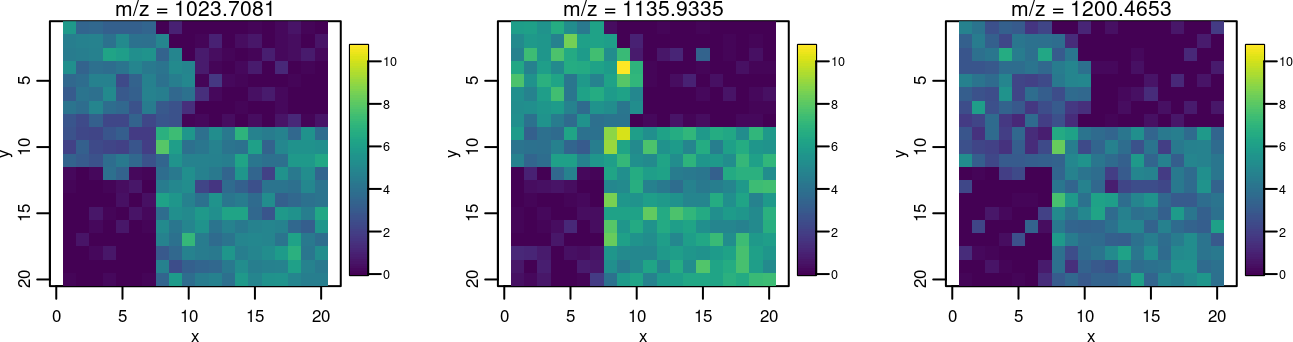# 3 Image segmentation

Segmentation (clustering) a dataset is a useful way to summarize an MS imaging experiment and discover regions of interest within the sample.

## 3.1 Spatial shrunken centroids clustering

Spatially-aware nearest shrunken centroids clustering allows simultaneous image segmentation and feature selection.

A smoothing radius `r`, initial number of clusters `k`, and sparsity parameters `s` must be provided.

The larger the sparsity parameter `s`, the fewer mass features will contribute to the segmentation.

Spatial shrunken centroids may result in fewer clusters than the initial number of clusters `k`, so it is recommended to use a value for `k` that is larger than the expected number of clusters, and allow the method to automatically choose the number of clusters.

``````ssc <- spatialShrunkenCentroids(mse, r=1, k=5, s=c(0,3,6,9))

summary(ssc)``````
``````## Spatially-aware nearest shrunken centroids:
##
##  Segmentation / clustering
##  Method = gaussian
##  Distance = chebyshev
##
##   Radius (r) Init (k) Shrinkage (s) Classes Features/Class
## 1          1        5             0       4          10.00
## 2          1        5             3       3           9.67
## 3          1        5             6       3           8.33
## 4          1        5             9       3           6.33``````

Plotting the predicted cluster probabilities shows a clear segmentation into the ground truth image.

``image(ssc, model=list(s=9), values="probability")``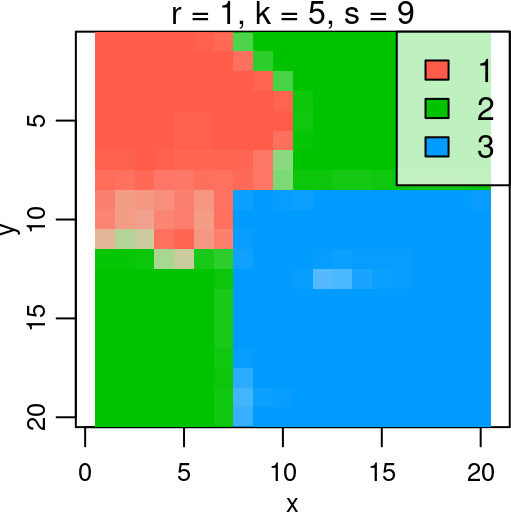Spatial shrunken centroids calculates t-statistics for each segment and each mass feature. These t-statistics a measure of the difference between the cluster center and the global mean.

``plot(ssc, model=list(s=9), values="statistic", lwd=2)``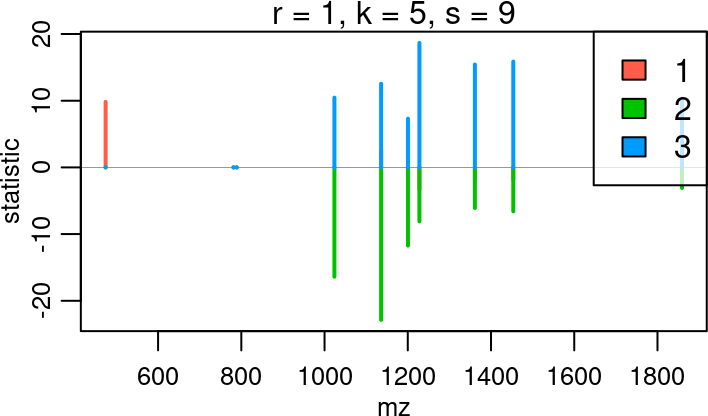Mass features with t-statistics of zero do not contribute to the segmentation. The sign of the t-statistic indicates whether the mass feature is over- or under-expressed in the given cluster relative to the global mean.

Use `topFeatures()` to rank mass features by t-statistic.

``````ssc_top <- topFeatures(ssc, model=list(s=9), class == 1)
ssc_top``````
``````## Top-ranked features:
##           mz   circle   square r k s class   centers statistic
## 1   473.9206 2.340799 0.000000 1 5 9     1 2.0820397  9.820019
## 2  1135.9335 2.434873 3.985370 1 5 9     1 4.1257736  2.689700
## 3   781.2367 1.392247 0.000000 1 5 9     1 0.4872447  0.000000
## 4   788.8633 1.542205 0.000000 1 5 9     1 0.5256366  0.000000
## 5  1023.7081 2.011661 4.063644 1 5 9     1 2.7843207  0.000000
## 6  1200.4653 2.219637 4.166854 1 5 9     1 2.4806421  0.000000
## 7  1858.8985 0.000000 3.970513 1 5 9     1 1.2799704  0.000000
## 8  1453.5096 0.000000 4.187344 1 5 9     1 1.3757443 -1.826775
## 9  1361.2682 0.000000 4.259568 1 5 9     1 1.3131620 -1.889194
## 10 1227.9380 0.000000 4.039750 1 5 9     1 1.2503541 -3.318708``````

## 3.2 Spatial Dirichlet Gaussian mixture modeling

Spatially-aware Dirichlet Gaussian mixture models (spatial-DGMM) is a method of image segmentation applied to each mass feature individually, rather than the dataset as a whole.

This is useful for summarizing molecular ion images, and for discovering structures that clustering using all mass features together may miss.

``````dgmm <- spatialDGMM(mse, r=1, k=5, method="adaptive")

summary(dgmm)``````
``````## Spatially-aware Dirichlet Gaussian mixture models:
##
##  Segmentation on 1 group: run0
##  Distance = chebyshev
##
##    Radius (r) Init (k) Feature Classes/Group
## 1           1        5       1             2
## 2           1        5       2             2
## 3           1        5       3             1
## 4           1        5       4             3
## 5           1        5       5             3
## 6           1        5       6             3
## 7           1        5       7             2
## 8           1        5       8             2
## 9           1        5       9             2
## 10          1        5      10             2``````

A different segmentation is fit for each mass feature.

``image(dgmm, model=list(feature=c(1,4,7)), layout=c(1,3))``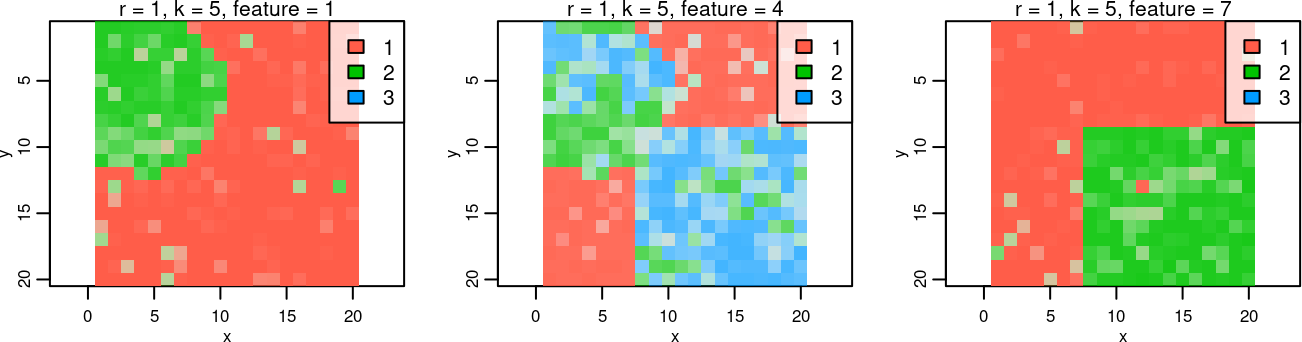Each image is modeled as a mixture of Gaussian distributions.

``plot(dgmm, model=list(feature=c(1,4,7)), layout=c(1,3))``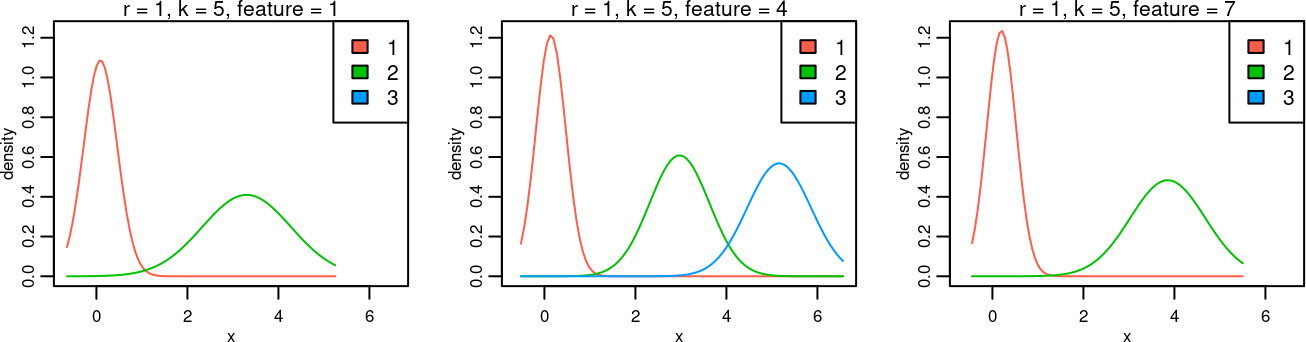Spatial-DGMM segmentations can be especially useful for finding mass features colocalized with a region-of-interest.

When applied to a `SpatialDGMM` object, `colocalize()` is able to use match scores that can have a higher specificity than using Pearson correlation on the raw ion images.

``````coloc2 <- colocalized(dgmm, mse\$square)
subset(coloc2, select=c(-r, -k, -group))``````
``````## Colocalized features:
##           mz   circle   square feature class    Mscore        M1        M2
## 1  1361.2682 0.000000 4.259568       8     2 0.9810127 0.9935897 0.9872611
## 2  1227.9380 0.000000 4.039750       7     2 0.9687500 0.9935897 0.9748428
## 3  1453.5096 0.000000 4.187344       9     2 0.9509202 0.9935897 0.9567901
## 4  1858.8985 0.000000 3.970513      10     2 0.9263804 0.9679487 0.9556962
## 5  1023.7081 2.011661 4.063644       4     3 0.6269430 0.7756410 0.7658228
## 6  1200.4653 2.219637 4.166854       6     3 0.5423729 0.6153846 0.8205128
## 7   473.9206 2.340799 0.000000       1     1 0.4983389 0.9615385 0.5084746
## 8  1135.9335 2.434873 3.985370       5     3 0.4352332 0.5384615 0.6942149
## 9   781.2367 1.392247 0.000000       2     1 0.4303797 0.8717949 0.4594595
## 10  788.8633 1.542205 0.000000       3     1 0.3900000 1.0000000 0.3900000``````

# 4 Classification and cross-validation

Classification of pixels into different known classes (e.g., cancer vs normal) based on the mass spectra is a common application for MS imaging.

``````set.seed(2020)
mse2 <- simulateImage(preset=7, npeaks=10, dim=c(10,10), sdnoise=0.5,
nruns=3, peakdiff=2, representation="centroid")

class <- makeFactor(A=mse2\$circleA, B=mse2\$circleB)

image(mse2, class ~ x * y, key=TRUE, layout=c(1,3))``````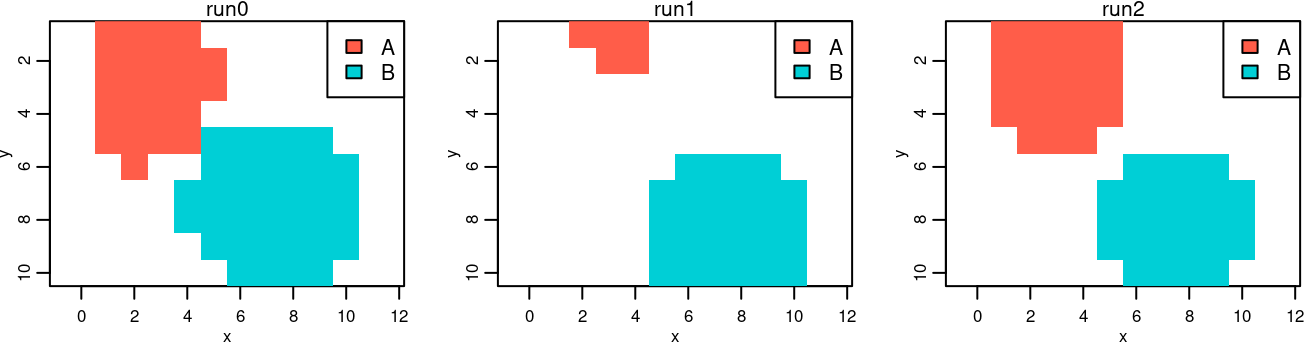``image(mse2, feature=1, layout=c(1,3))``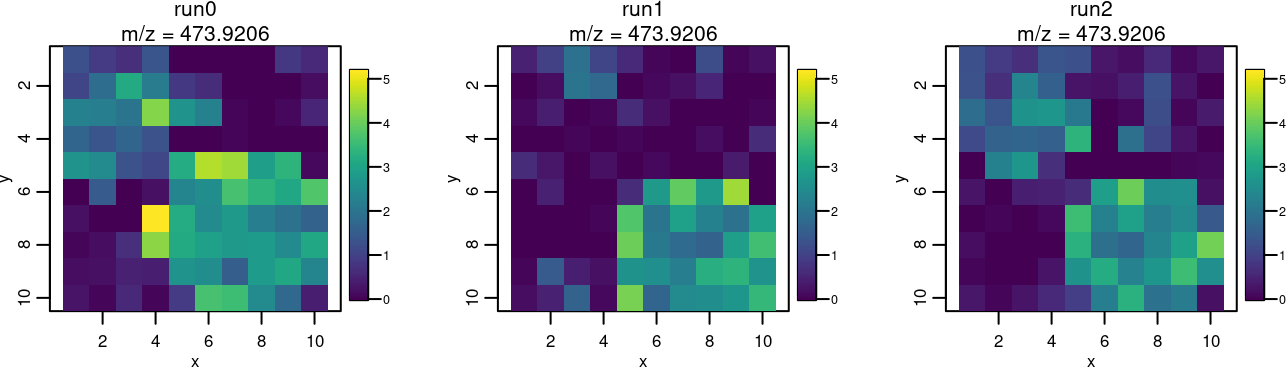When performing classification, it is important to use cross-validation so that reported accuracies are not overly optimistic.

We strongly recomend making sure that all spectra from the same experiment run belong to the same fold, to reduce predictive bias due to run effects.

## 4.1 Projection to latent structures (PLS)

Projection to latent structures (PLS), also called partial least squares, is a supervised dimension reduction technique. It can be thought of as being similar to PCA, but for classification or regression.

``````cv_pls <- crossValidate(mse2, .y=class, .fun=PLS, ncomp=1:5, .fold=run(mse2))

summary(cv_pls)``````
``````## Cross validation:
##
##  Classification on 2 classes: A B
##  Summarized 3 folds: run0 run1 run2
##
##   ncomp  Accuracy Sensitivity Specificity
## 1     1 0.6608485   0.0000000   1.0000000
## 2     2 0.8363402   0.6579710   0.9538462
## 3     3 0.9247009   0.8405797   0.9871795
## 4     4 0.9383064   0.8840580   0.9809524
## 5     5 0.9325592   0.8985507   0.9619048``````

We can see that using 3 PLS components produces the best cross-validated accuracy.

``````pls <- PLS(mse2, y=class, ncomp=3)

summary(pls)``````
``````## Projection to latent components:
##
##  Classification on 2 classes: A B
##  Method = pls
##
##   Number of Components  Accuracy Sensitivity Specificity
## 1                    3 0.9285714   0.8235294    0.988764``````

We can plot the fitted values to visualize the prediction.

``image(pls, values="fitted", layout=c(1,3))``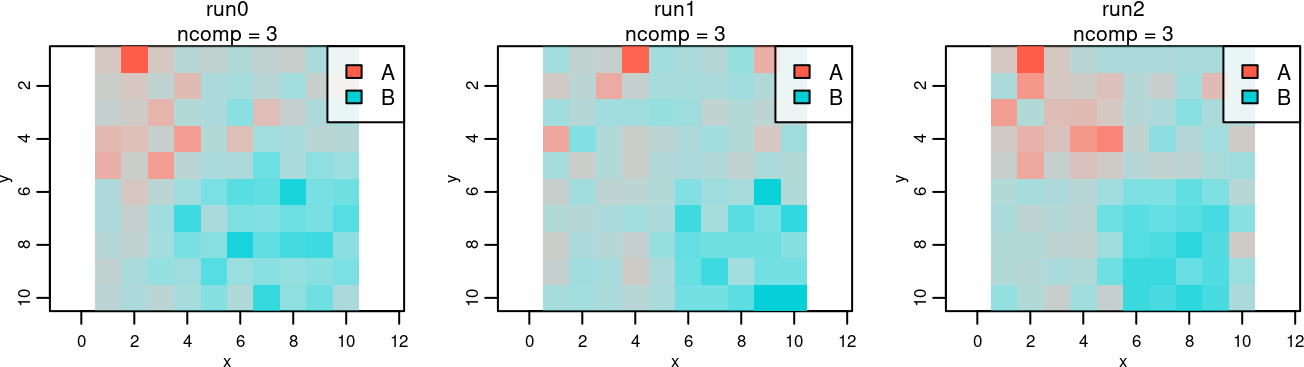The PLS regression coefficients can be used to select influential features.

``plot(pls, values="coefficients", lwd=2)``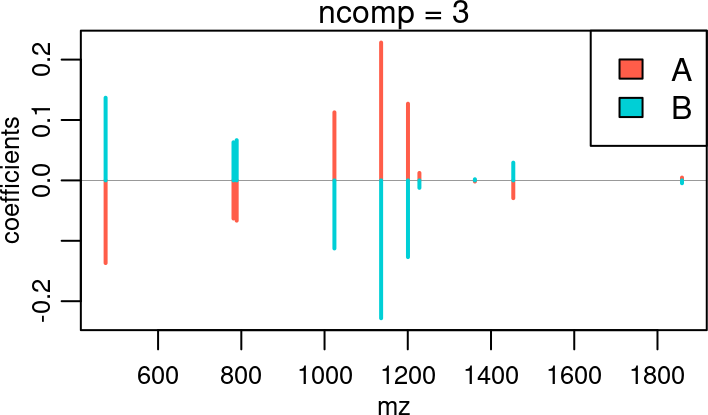Like PCA, it can be useful to plot the PLS scores against each other to visualize the separation between classes.

``````pls_scores <- DataFrame(resultData(pls, 1, "scores"))

plot(pls_scores, C1 ~ C2, groups=class, pch=20)``````